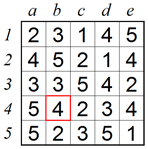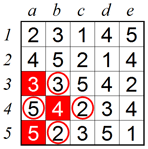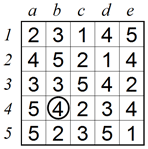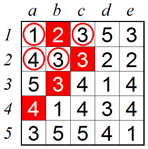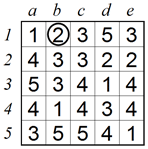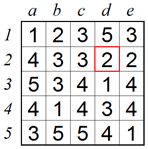﻿ Hitori решение
 ВходРегистрация# Hitori решение

Hitori is a number-elimination puzzle based on a square grid filled with numbers. The object is to shade squares so that no number appears in a row or column more than once. In addition, shaded (black) squares do not touch each other vertically or horizontally and all un-shaded (white) squares create a single continuous area when the puzzle is completed. In this example we have a 5x5 Hitori puzzle with five columns marked a through e, and five rows marked 1 through 5. It is clear that some rows and columns have the same numbers more than once and some numbers must be shaded… but how?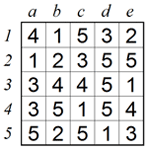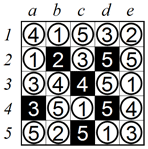## Starting techniques

The easiest way to begin a Hitori puzzle is to scan rows and columns using the starting techniques. After finding and solving these first clues, proceed to the next techniques to continue solving the puzzle. Here are some ways of using the starting techniques:

#### 1. Searching for adjacent triplets:

The middle square of an adjacent triplet must always be un-shaded. Let’s look at column d which has a triplet of adjacent 5s. The first rule of Hitori says the same number cannot appear more than once in a row or a column so we need to shade some of the 5s. If we shade all three we violate the second rule which says shaded squares must not touch each other vertically or horizontally. This violation will also occur if we shade just the two top 5s or the two bottom 5s. So the only possibility left is to shade the top and bottom 5s, and mark a circle around the middle 5 to indicate it must remain un-shaded.#### 2. Square between a pair:

A square between a pair of same-numbers must be un-shaded. In this example, the 4 in row 4 is placed between a pair of 2s. The second rule of Hitori says shaded squares must not touch each other vertically or horizontally implying that a shaded square is always surrounded by four un-shaded squares (or less, depending on whether it is located next to the edge or in a corner). If we try shading the 4 then the adjacent 2s must be un-shaded which is not permitted according to the first rule. Therefore the 4 in square b4 is marked with a circle to indicate it is un-shaded.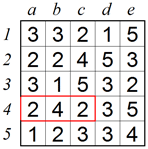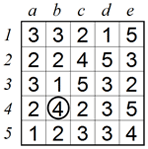#### 3. Pair induction:

Another interesting starting technique is pair induction, where a row or column contains three same-numbers but only two of them are an adjacent pair. In row 4 of this example there are three 5s, two of which are an adjacent pair and one which is single. If the single 5 is un-shaded, then according to the first rule of Hitori the adjacent pair of 5s must be shaded. But this would violate the second rule which says shaded squares must not touch each other vertically or horizontally. Therefore the 5 in square b4 must be shaded.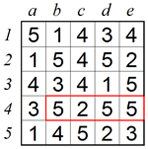## Basic techniques

After solving the first clues using starting techniques there is usually a straightforward phase of shading and un-shading squares according to the three rules of Hitori. However, some hard puzzles may require the use of corner techniques or even advanced techniques right away. Here are some ways of using the basic techniques:

#### 1. Shading squares in rows and columns:

The first rule of Hitori says same number cannot appear more than once in a row or a column. In this example square b5 contains an un-shaded 2 from the previous solution steps of the puzzle. This means the 2 in square b2 must be shaded to avoid conflict with this rule.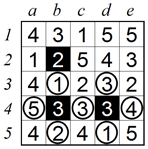The second rule of Hitori says shaded squares must not touch each other vertically or horizontally. In this example there are two shaded squares from the previous solution steps of the puzzle. This means we can un-shade all adjacent squares by marking them with circles.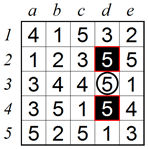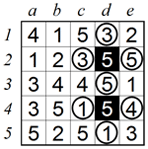#### 3. Un-shading squares to avoid partitions:

The third rule of Hitori says un-shaded squares must create a single continuous area. In this example squares b1, c2, and d3 form a wall of shaded squares. This means squares e2 and e4 must be un-shaded to avoid partitioning the un-shaded area.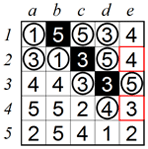## Corner techniques

Hitori puzzles many times create interesting situations in the corners, which require more advanced techniques to solve. It is always useful to take a close look at all four corners to see if these situations occur in the puzzle. Here are some ways of using the corner techniques:

#### Corner technique 1:

If square a1 is un-shaded then the two adjacent squares in b1 and a2 must be shaded according to the first rule of Hitori. However, by shading squares b1 and a2, a wall is formed in the corner which partitions the un-shaded square a1 from the rest of the un-shaded area. Therefore square a1 must be shaded, and we can also un-shade squares b1 and a2.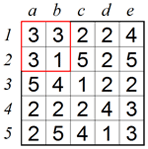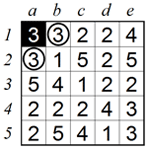#### Corner technique 2:

Here is another special corner situation. From the first rule, either square a1 or a2 must be shaded. If we shade square a2 then square b2 must be un-shaded, which in turn means square b1 must be shaded as well. We now have both a2 and b1 shaded which partitions the un-shaded square a1 from the rest of the puzzle. Therefore square a1 must be shaded, which leads to the un-shading of a2 and b1 and the shading of b2.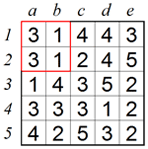The techniques described so far won’t be enough to solve medium and hard puzzles. For this you will need wits and advanced techniques to work out many special and interesting logic situations. Most advanced techniques are done by a process of assumption and conflict, where a square is assumed to be shaded (or un-shaded) and this assumption is proven wrong in the next step or two using logic. Here are some examples of advanced techniques to solve special situations. You will develop many more advanced techniques when you solve Hitori puzzles by yourself:

If we un-shade square a5, then squares a3 and c5 must be shaded which creates a partition wall and violation of the third rule of Hitori. Therefore square a5 must be shaded.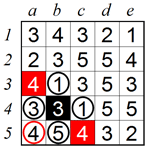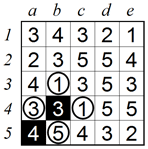If we shade square c5, then a partition violation will occur when the 3 in square e5 or a5 is shaded. Therefore square c5 must be un-shaded.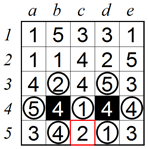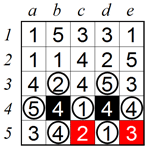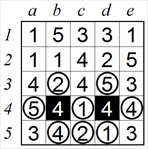If we shade square b4, then squares b3 and a4 must be un-shaded. This means squares a3 and a5 must be shaded, which will cause partitioning of square a4. Therefore square b4 must be un-shaded.